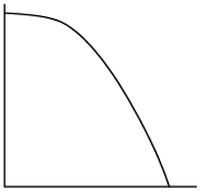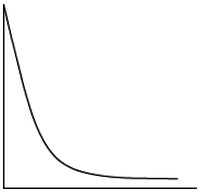# Words - Tables - Graphs

Alignments to Content Standards: F-IF.B.4

Below are 4 verbal descriptions, 3 graphs, and 3 tables of values. Match each of the following descriptions with an appropriate graph and table of values. Create the missing graph and the missing table of values.

1. The weight of your jumbo box of cereal decreases by an equal amount every week.

2. The value of the bicycle depreciated rapidly at first, but its value declined more slowly as time went on.

3. The tennis ball is dropped off the roof of a skyscraper.

4. For a while it looked like the decline in profits was slowing down, but then they began declining more rapidly.

A.

 x 0 1 2 3 4 5 y 400 384 336 256 144 0

B.

 x 0 1 2 3 4 5 y 400 184 98 63 49 43

C.

 x 0 1 2 3 4 5 y 400 253 218 216 181 34
1.2.3.## IM Commentary

The fourth description can be challenging for students. The teacher could change the quantity to make it easier for students to understand or decide to leave out this part, if necessary.

## Solution

Description 1 indicates a constant rate of change. The graph must be (III), since the line has a constant rate of change. The table must show a change for each time interval of 1 as the same. In the table shown below, the change for each time interval of 1 is 80.

 x 0 1 2 3 4 5 y 400 320 240 160 80 0

(x represents time in weeks. y represents weight, probably in grams, at least that would fit best with the numbers.)

Description 2 indicates a rapid change and then a slow change. The table must be (B), since the change in each interval of 1 decreases as x increases. The graph must drop quickly and then slow, as shown below.(x represents time, in years (makes most sense) y represents value in dollars (could be other currencies))

Description 3 indicates a slow change and then a rapid change. The graph must be (I), since it decreases slowly at first and then drops quickly. The table must be (A), since the change in each interval of 1 increases as x increases.

(x represents height in meters of feet y represents time, in seconds (makes most sense))

Description 4 indicates a quick change then a slow change and then a quick change. The graph must be (II), since the graph drops quickly, then steadies for a short while, and then drops quickly again. The table must be (C), since the change in each interval of 1 is large, then small, and then large again.

(x represents time, the units are not given and could vary, i.e. days, weeks, years are all possible. y represents profit in dollars, (could be different currency or method to measure profits))